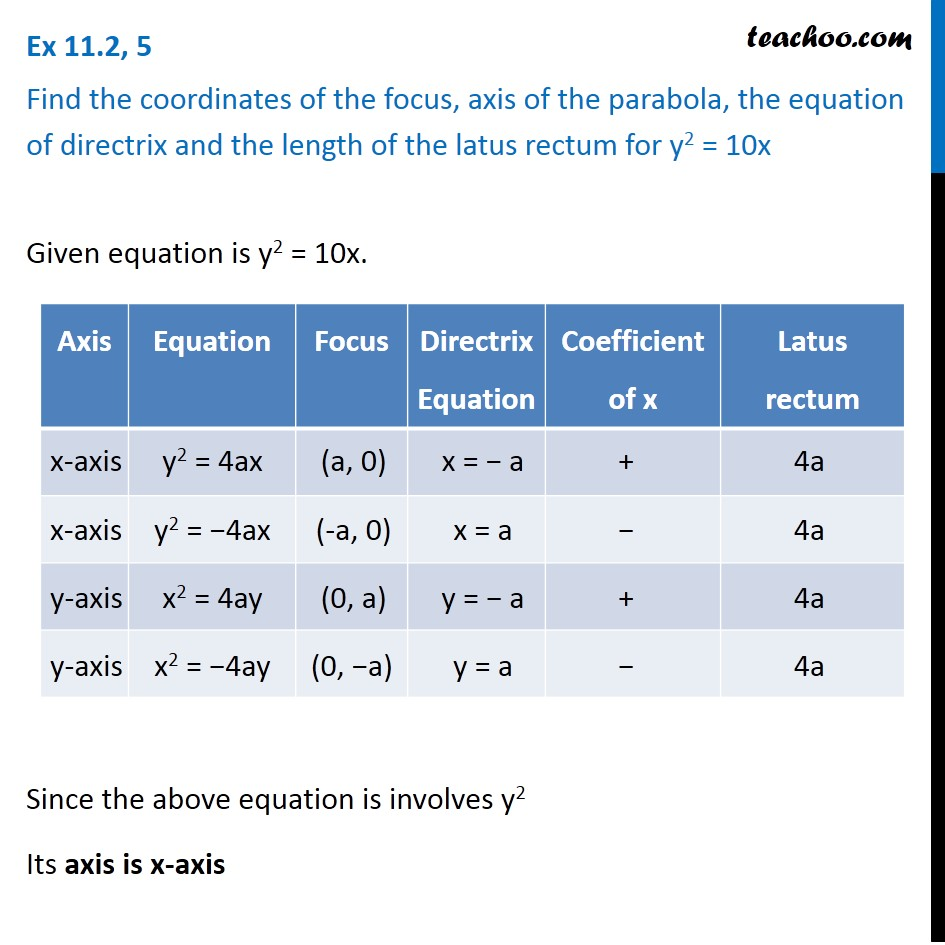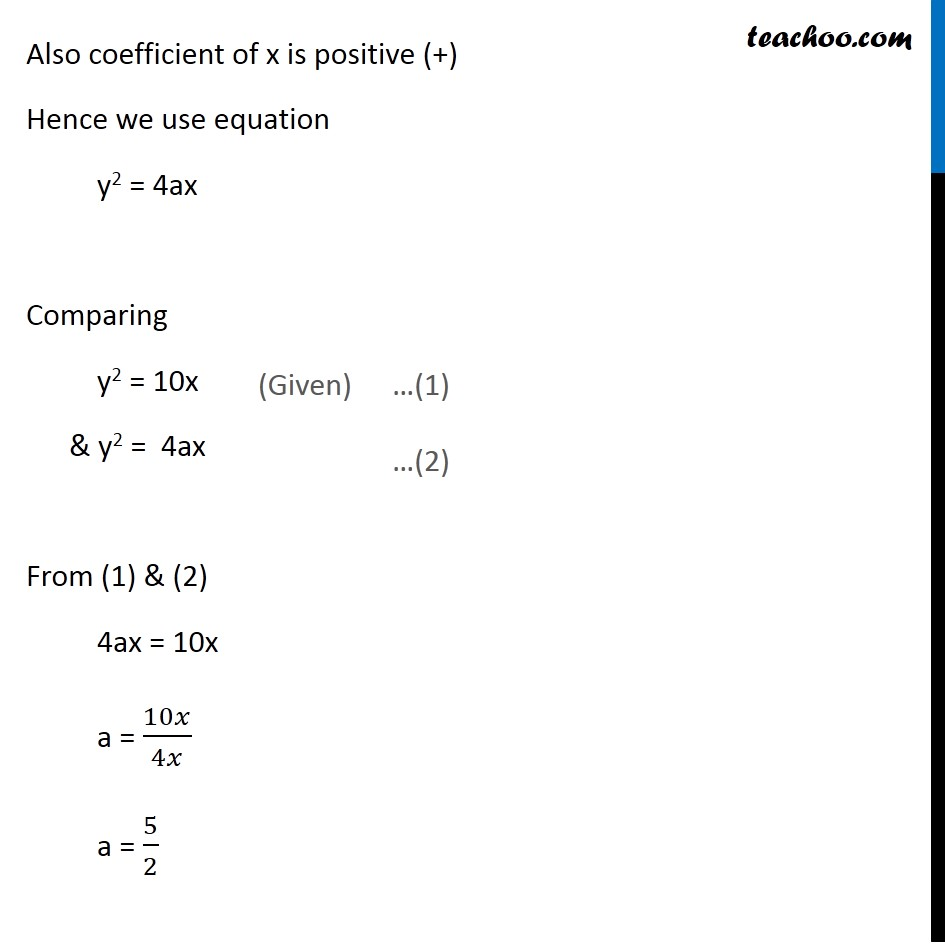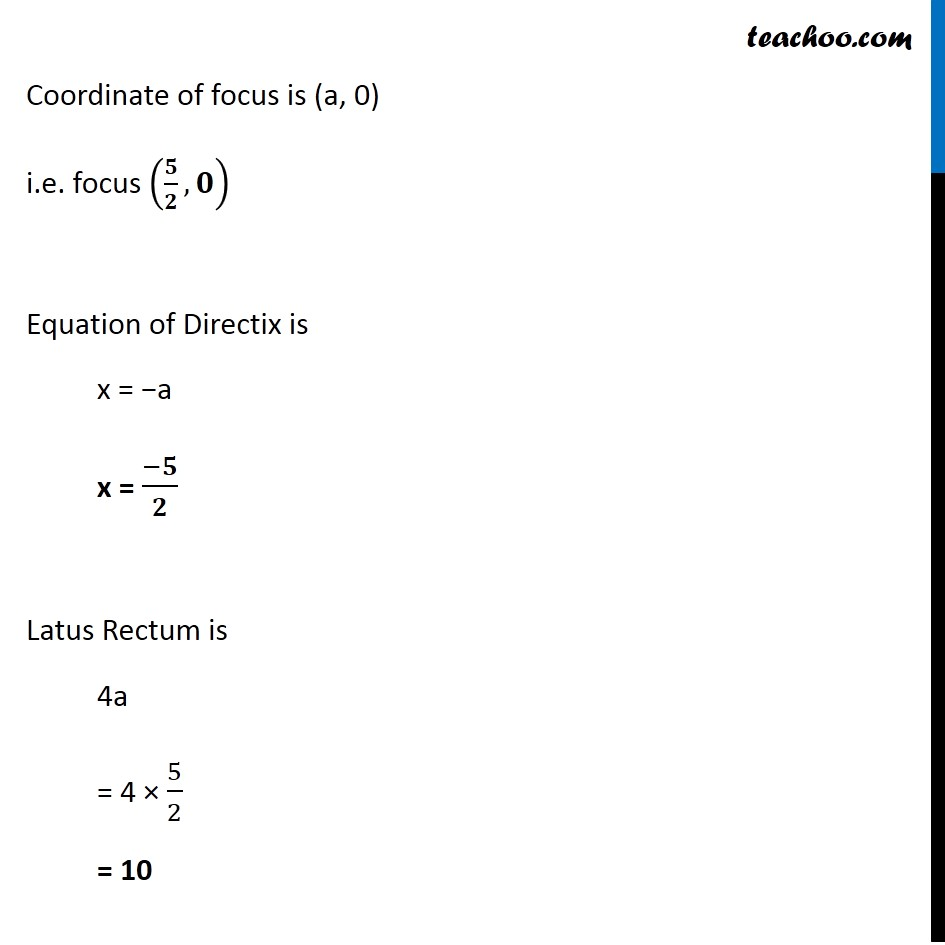Ex 10.2

Chapter 10 Class 11 Conic Sections
Serial order wiseLearn in your speed, with individual attention - Teachoo Maths 1-on-1 Class

### Transcript

Ex 10.2, 5 Find the coordinates of the focus, axis of the parabola, the equation of directrix and the length of the latus rectum for y2 = 10x Given equation is y2 = 10x. Since the above equation is involves y2 Its axis is x-axis Also coefficient of x is positive (+) Hence we use equation y2 = 4ax Comparing y2 = 10x & y2 = 4ax From (1) & (2) 4ax = 10x a = 10𝑥/4𝑥 a = 5/2 Coordinate of focus is (a, 0) i.e. focus (𝟓/𝟐,𝟎) Equation of Directix is x = −a x = (−𝟓)/𝟐 Latus Rectum is 4a = 4 × 5/2 = 10